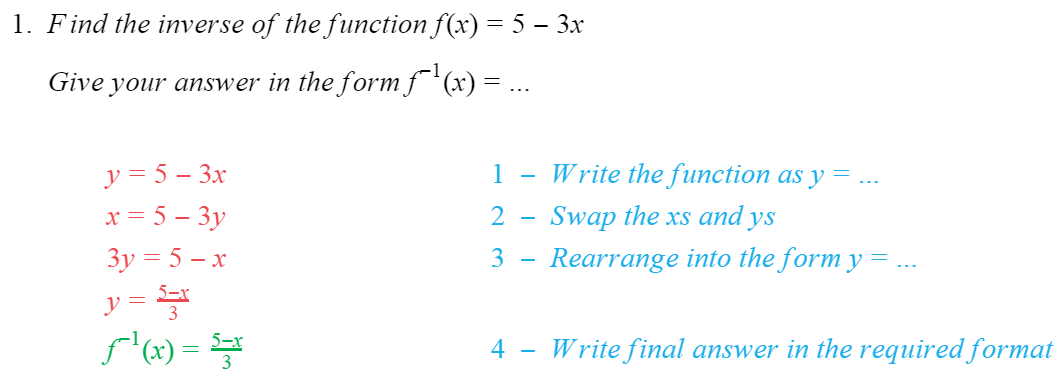# Edexcel IGCSE Maths 复习笔记 3.2.3 Inverse Functions

Edexcel IGCSE Maths 复习笔记 3.2.3 Inverse Functions

#### What is an inverse function?

• An inverse function does the exact opposite of the function it came from
• Eg. if the function “doubles the number and adds 1”then its inverse will “subtract 1 and halve the result”
• It is the INVERSE operations in the reverse order

#### What do inverse functions look like?

• An inverse function f-1 can be written as:f-1(x) = … or f-1 : x ↦ …
• Eg. if f(x) = 2x + 1 its inverse can be written as:f-1(x) = x – 1 / 2   or   f-1: x ↦ x – 1 / 2

#### What do you find an inverse function?

1. Write the function in the form y=…
2. SWAP the xs and ys to get x=…
3. REARRANGE to give y=… again
4. Write as f-1(x) = … (or f-1 : x ↦ …)
• Eg. if f(x)=2x+1 its inverse can be found as follows …y= 2x + 1 1   –   1. Write the function as y=…x = 2y + 1 2  –   2. Swap the xs and ysx – 1= 2y 3   –   3. Rearrange into the form y=… (or …=y)

x – 1 / 2 = yf-1(x) = x – 1 / 2  – 4. Write as f-1(x)=…

#### Worked Example